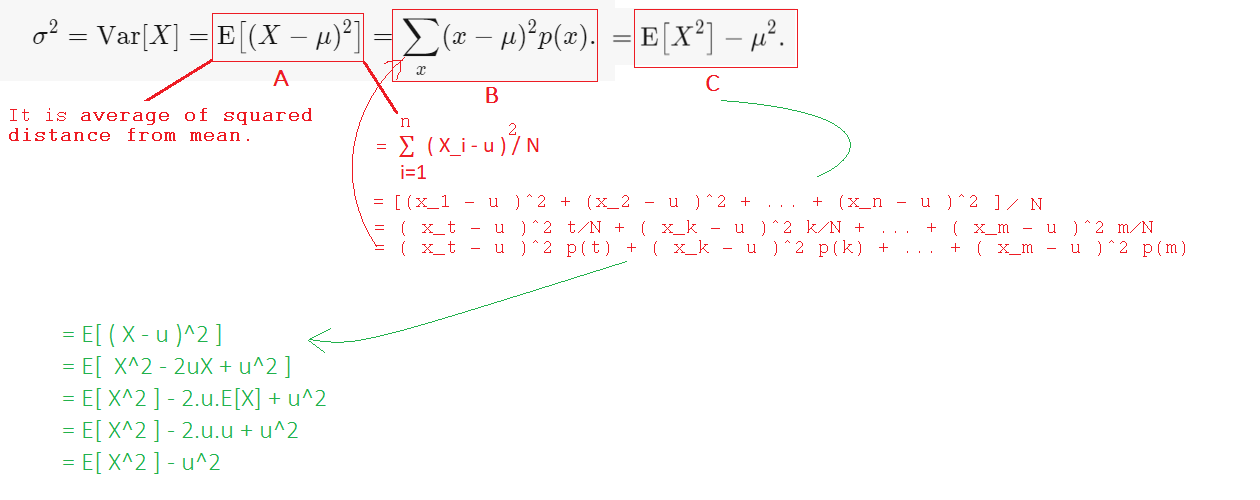# Variance, in Words

Let’s assume L is a population set. Variance of L tells, how much datapoints of L are far from L’s mean(u).Definition of Variance

Also,

1. Why Var[c] = 0 ?

Let’s recall, how we denoted variance. It is Var[X] where X is a random variable i.e. X can take different values based on some events. But ‘c’ is a constant i.e. E[c] = c.

So Var[c] = 0.

2. How variacne acts when each point of L is multipled by some constant ‘c’ ?

Let’s assume ‘u_c’ is modified mean of set L after multiplying ‘c’ with each point in L.

Now,

So, Var[cX]= c² * Var[X]

3. How variance acts when each point of L is added by some constant ‘c’ ?

So, Var[cX]= Var[X]

4. Var[aX+b] = ?

So, Var[aX+b] = a² . Var[X].

Also we can think like ( aX+b ) as ( X* + b ) then Var[X*+b] = Var[X*] = Var[aX] = a².Var[X]

5. Var[X+Y] = Var[X] + Var[Y] + 2.XY.Cov(X,Y) ?

6.

References:

 Brilliant: https://brilliant.org/wiki/variance-definition/

NLP Enthusiast